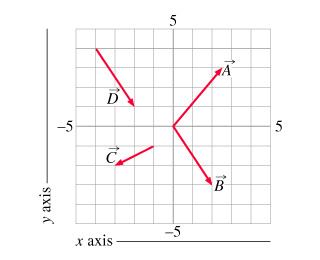# Problem: The following questions will ask you to give both components of vectors using the ordered pairs method. In this method, the x component is written first, followed by a comma, and then the y component. For example, the components of A_vec would be written 2.5,3 in ordered pair notation.The answers below are all integers, so estimate the components to the nearest whole number.In ordered pair notation, write down the components of vector B_vec.Express your answers to the nearest integer.B_x, B_y =

###### FREE Expert Solution

The tail of B is at x = 0 and  y = 0. Its head is at x =2 and y = -3.

85% (168 ratings)###### Problem Details

The following questions will ask you to give both components of vectors using the ordered pairs method. In this method, the x component is written first, followed by a comma, and then the y component. For example, the components of A_vec would be written 2.5,3 in ordered pair notation.

The answers below are all integers, so estimate the components to the nearest whole number.

In ordered pair notation, write down the components of vector B_vec.